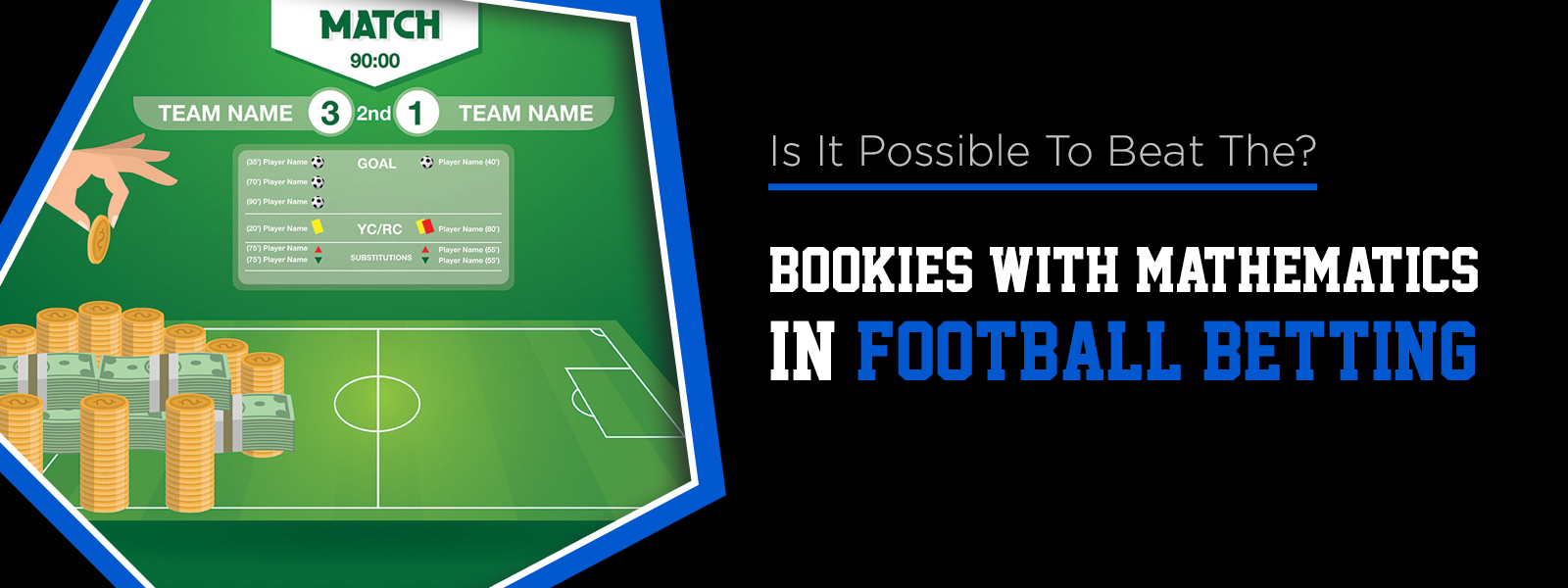X
Highlight
Is It Possible To Beat The Bookies With Mathematics In Football Betting?## Beat The Bookies With Mathematics

Usually, novice punters think they can beat the bookies based on their sports knowledge and reading soccer news. What they don't know is that soccer betting is more about mathematics than soccer itself. In fact, advanced bettors use probabilities and stats as their primary tools to win by betting. There are many excellent soccer betting systems, and all of them have mathematics as their base.

On the other hand, we have the stats and trends as another mathematical tool widely used in soccer betting. Usually, they are used to know the betting systems' effectiveness. Besides, they allow us to estimate better the occurring probability of a match's possible result.

### Football Betting and Probabilities

We cannot predict with total accuracy the occurring probability of a sporting event. For this reason, bookmakers use systems and statistical data to estimate probabilities for each event. Thus, we must remember that the events' occurring probability is predefined by bookies. Our goal as bettors is to estimate these probabilities better. In this sense, information and mathematical analysis are crucial.

To achieve this, we must convert the sports bookie's odds into probabilities through the following formula:

Probability = (1 / bookie's odd) x 100

This means that the probability (in percentage) assigned by the bookmaker is the odd's inverse multiplied by 100. This probability is approximate since always the bookmakers include in the odds a small commission in their favor. The important thing is to know that through odds, we can see the probability of the sportsbook estimates for each possible outcome of a match.

### How To Win At Football Betting Using Mathematics

To win betting on soccer, we must find matches in which the probability estimated by us is higher than the bookmakers' probability. In this sense, you'll need to have enough information and to know well the leagues and teams in which you'll bet.

Let's take as an example a hypothetical match between Palmeiras and Gremio with the following odds:

• Gremio (away team) = 2.90 (the odd's implied probability is 34.5%)
• Draw = 4.40 (the odd's implied probability is 22.7%)
• Palmeiras (local) = 1.76 (the odd's implicit probability is 56.8%)

Suppose it is forecast that there will be rain during this match. So, you think this may favor Gremio. What you would do is estimating different probabilities than those the bookie expected. In this case, the bookmaker estimated a Gremio's winning probability equal to 34.5%. However, if you believe that, under these conditions, Gremio has greater possibilities, we will have what is called a value bet.

### Conclusion

There is no secret equation to predict the outcome of soccer games. Yet, to develop your own soccer results model, you need to use odds as a starting point. Through using the formula above, you should calculate the betting odds implicit probability every single time you place a bet. Then, estimate chances for each possible outcome and compare them with the bookie's expectations. Only bet if the probability you determine is higher than the bookmakers' implied probability.

Keeping this in mind, you're on your way to beat the bookies in the market– using mathematics like the pros!

Categories
tag
X